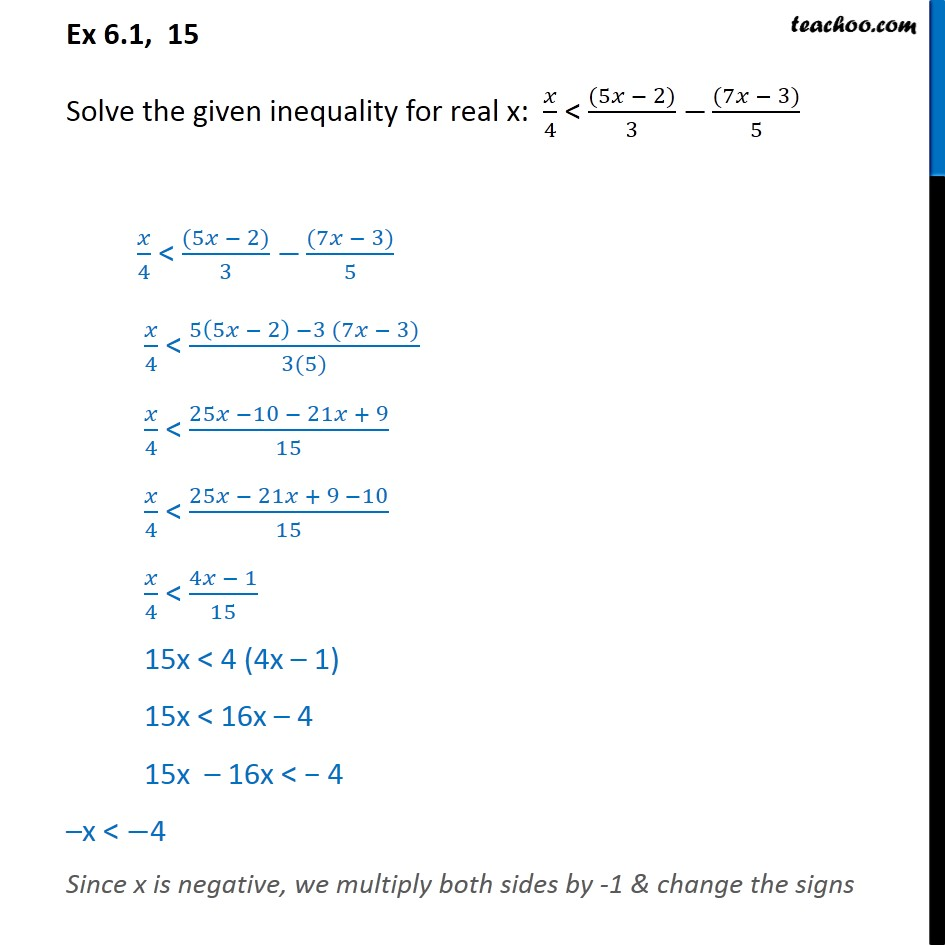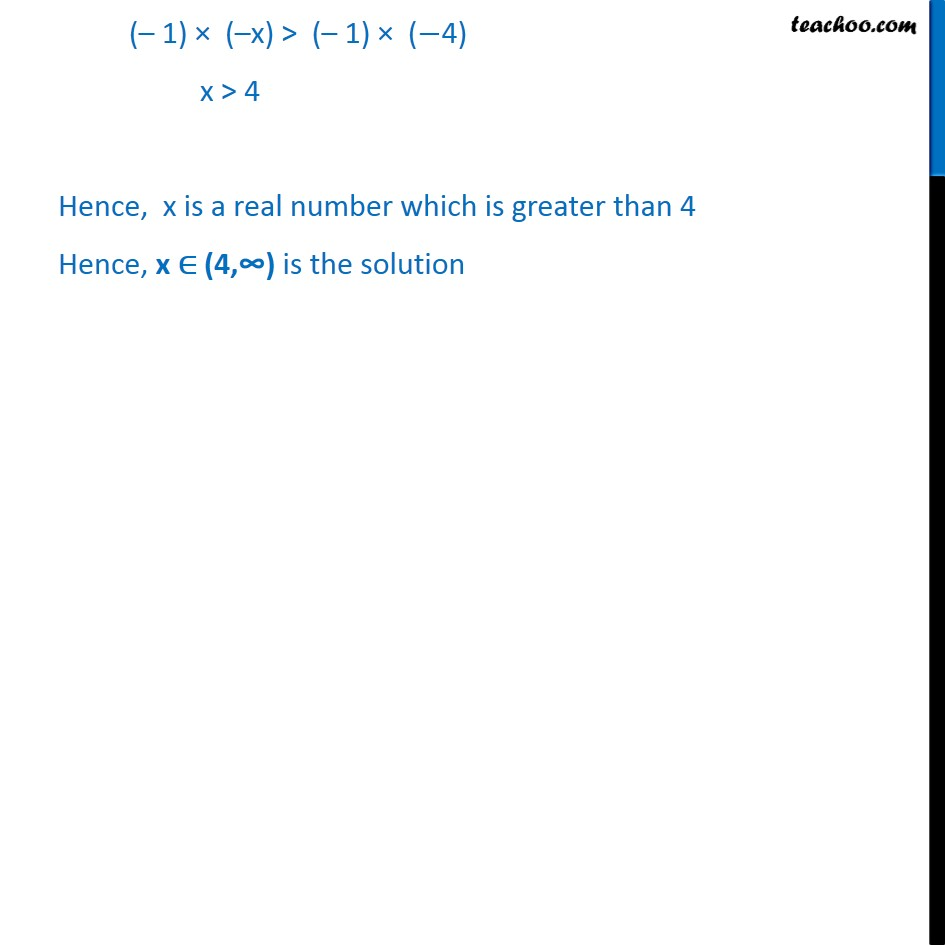Ex 6.1

Chapter 6 Class 11 Linear Inequalities
Serial order wiseMaths Crash Course - Live lectures + all videos + Real time Doubt solving!

### Transcript

Ex 6.1, 15 Solve the given inequality for real x: ﷐𝑥﷮4﷯ < ﷐(5𝑥 − 2)﷮3﷯ −﷐(7𝑥 − 3)﷮5﷯ ﷐𝑥﷮4﷯ < ﷐(5𝑥 − 2)﷮3﷯ −﷐(7𝑥 − 3)﷮5﷯ ﷐𝑥﷮4﷯ < ﷐5﷐5𝑥 − 2﷯ −3 (7𝑥 − 3)﷮3(5)﷯ ﷐𝑥﷮4﷯ < ﷐25𝑥 −10 − 21𝑥 + 9﷮15﷯ ﷐𝑥﷮4﷯ < ﷐25𝑥 − 21𝑥 + 9 −10﷮15﷯ ﷐𝑥﷮4﷯ < ﷐4𝑥 − 1﷮15﷯ 15x < 4 (4x – 1) 15x < 16x – 4 15x – 16x < − 4 –x < −4 Since x is negative, we multiply both sides by -1 & change the signs (– 1) × (–x) > (– 1) × (−4) x > 4 Hence, x is a real number which is greater than 4 Hence, x ∈ (4,𕔴 is the solution